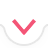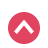# Properties

Quiz History

Select Topics
Domain

Range

Inverse

Parity
Start Quiz
Quiz Assignment

00:00:00Summary
Previous Question

Show Hints

3 / 3
Sorry, your browser does not support this application
x^2
x^{\msquare}
\frac{\msquare}{\msquare}
\sqrt{\square}
\nthroot[\msquare]{\square}
f(x)
\infty
\le
\ge
\mathrm{odd}
\mathrm{even}
\mathrm{neither\:even\:nor\:odd}
Let's Try Again :(
Try to simplify further
Thank you!

## Related Tutorials and Articles

Function Properties: Domain
Function Properties: Range Pt 1
Function Properties: Range Pt 2
Function Properties: Inverse
Function Properties: Parity
Start Quiz 00:00:00
Previous Question
1 / 10
x^2
x^{\msquare}
\frac{\msquare}{\msquare}
\sqrt{\square}
\nthroot[\msquare]{\square}
f(x)
\infty
\le
\ge
\mathrm{odd}
\mathrm{even}
\mathrm{neither\:even\:nor\:odd}
Finish Quiz

Symbolab

# Summary

Submit Assignment Start Over Back

# Summary

Start Again Back
Prev Student Next Student

in 01:12:13 hoursBack
Back

## Steps

Sorry, your browser does not support this application
Edit Quiz
Quiz Saved!# Crowns

You haven't collected any crowns yet

# Quizzes